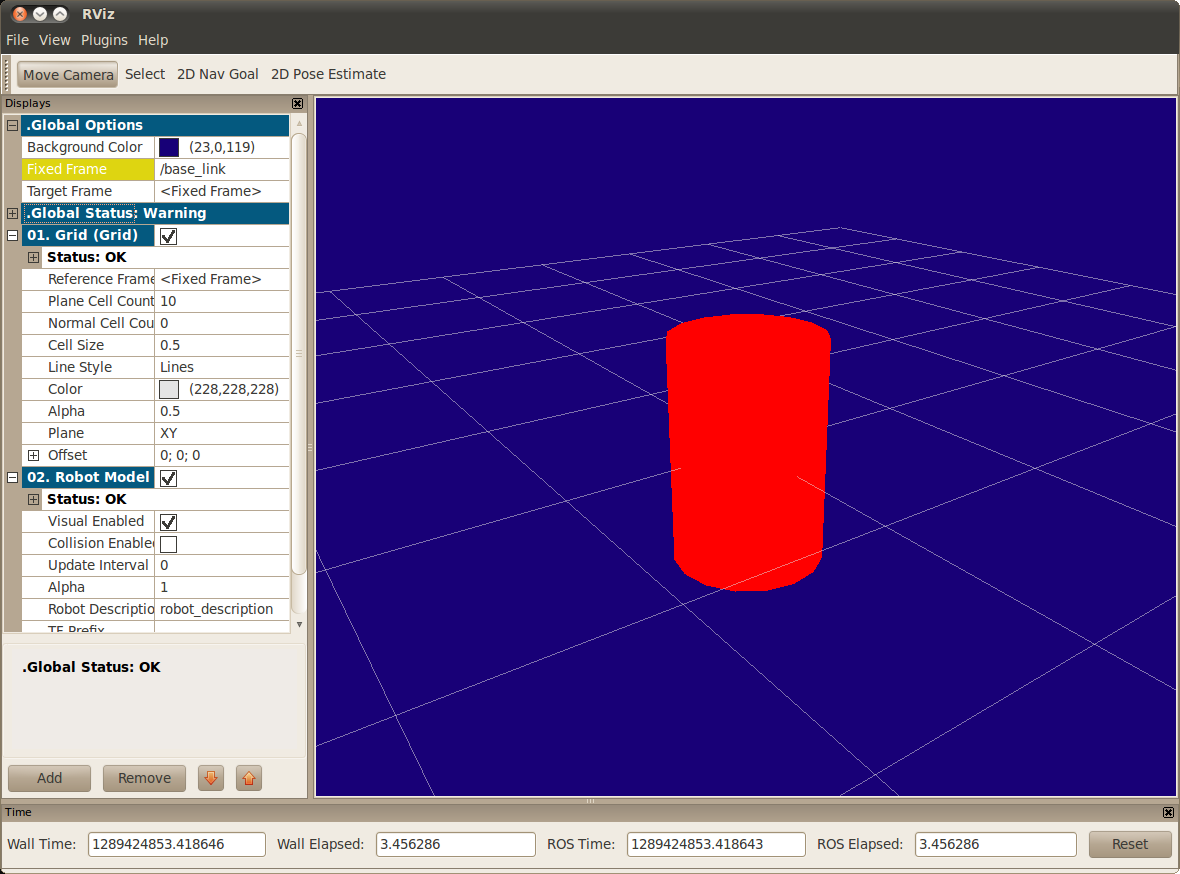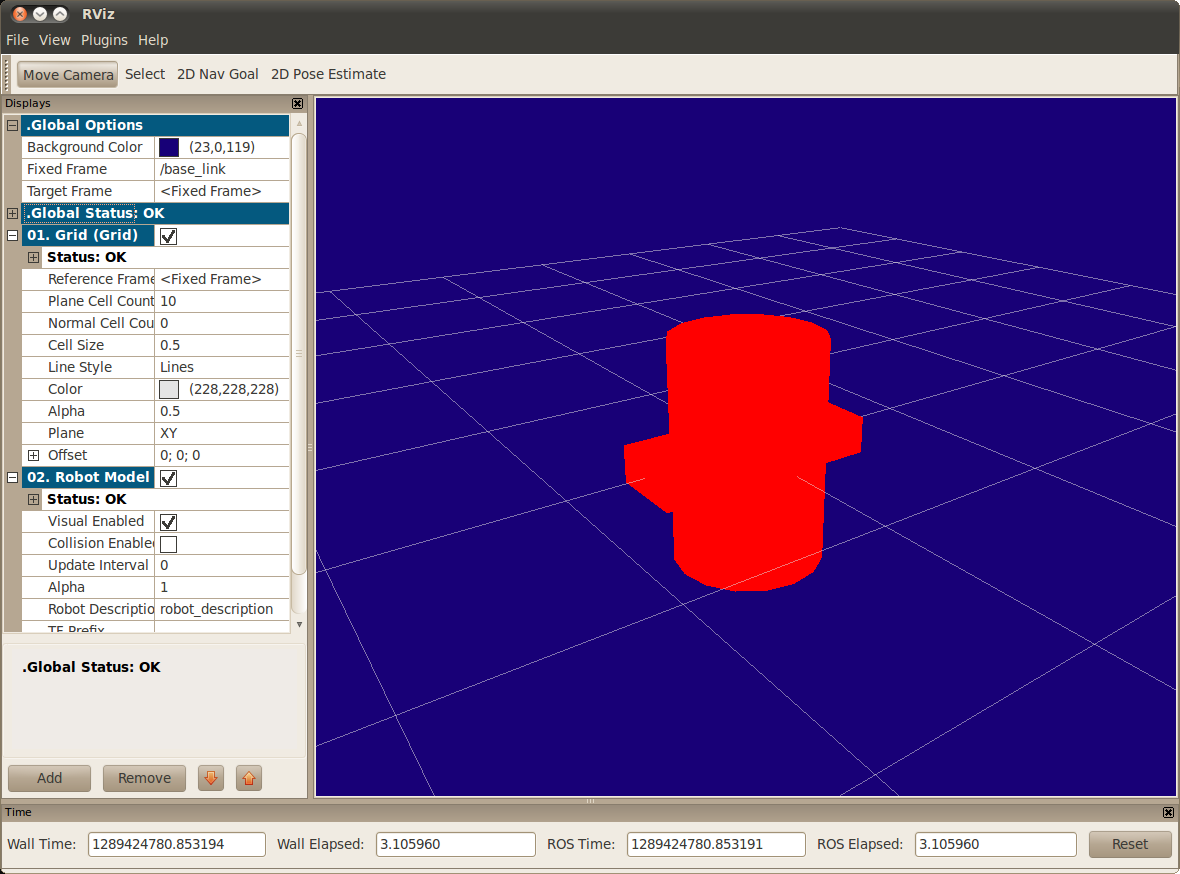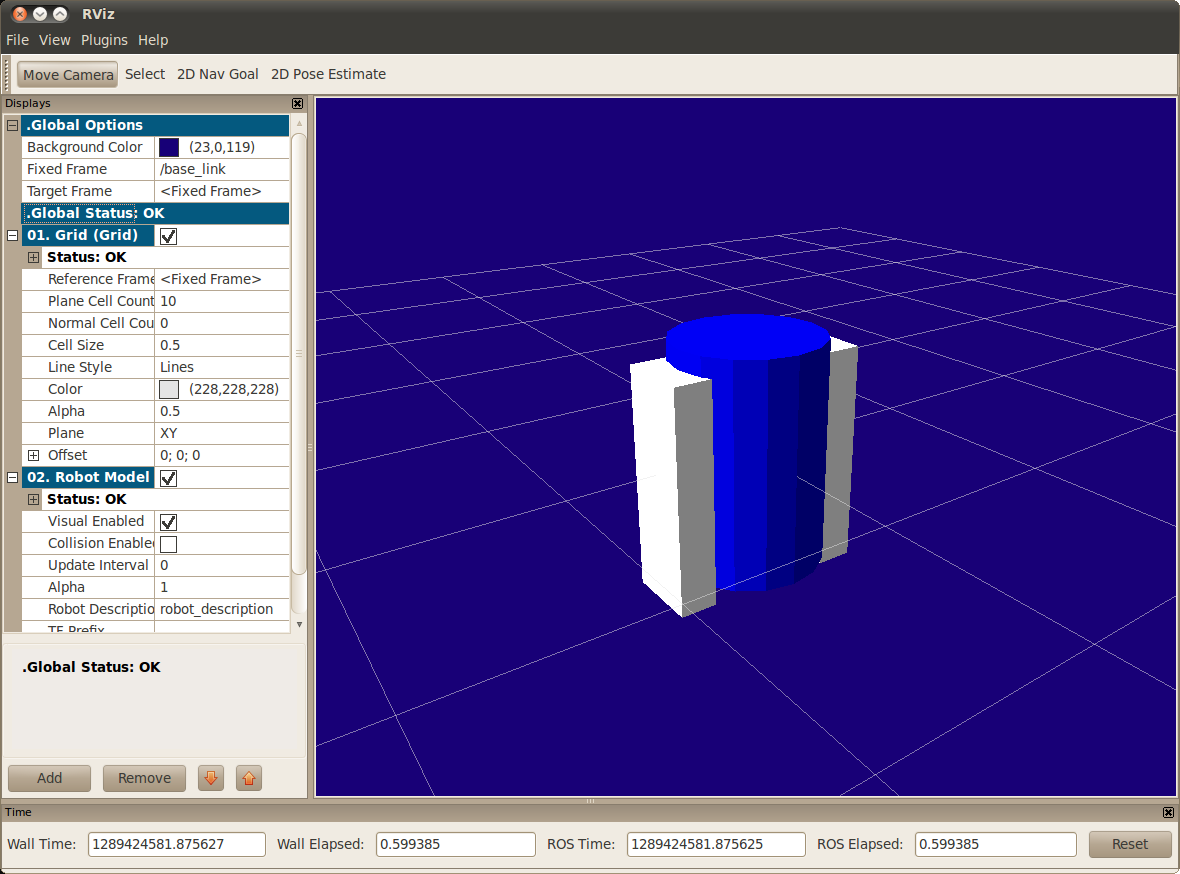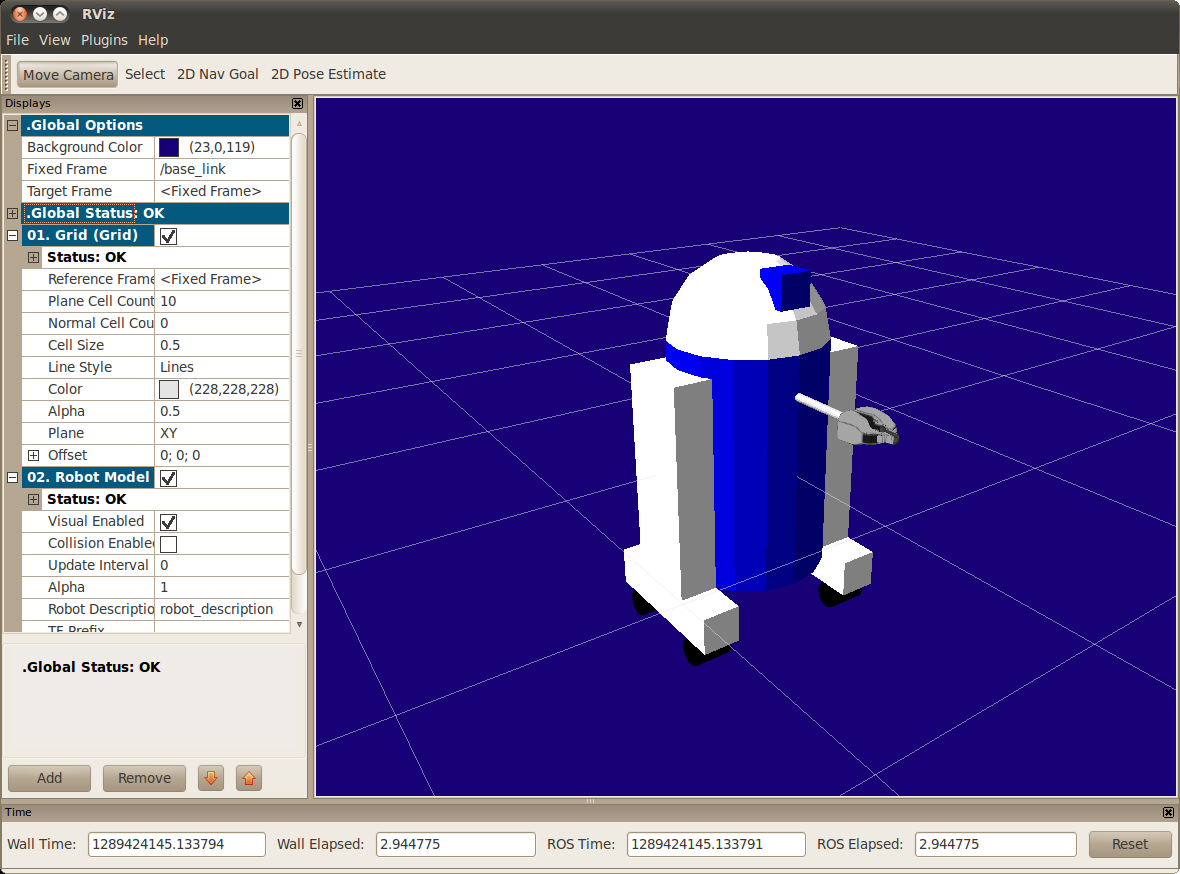Note: This tutorial assumes you know how to write well-formatted XML code.Please ask about problems and questions regarding this tutorial on answers.ros.org. Don't forget to include in your question the link to this page, the versions of your OS & ROS, and also add appropriate tags.

# Building a Visual Robot Model with URDF from Scratch

Description: Learn how to build a visual model of a robot that you can view in Rviz

Keywords: URDF

Tutorial Level: BEGINNER

Next Tutorial: Making the Model Move

In this tutorial, we’re going to build a visual model of a robot that vaguely looks like R2D2. In later tutorials, you’ll learn how to articulate the model, add in some physical properties, generate neater code with xacro and make it move in Gazebo. But for now, we’re going to focus on getting the visual geometry correct.

Before continuing, make sure you have the joint_state_publisher package installed. If you installed urdf_tutorial using apt-get, this should already be the case. If not, please update your installation to include that package (use rosdep to check).

All of the robot models mentioned in this tutorial (and the source files) can be found in the urdf_tutorial package.

## One Shape

First, we’re just going to explore one simple shape. Here’s about as simple as a urdf as you can make. Source

```   1 <?xml version="1.0"?>
2 <robot name="myfirst">
4     <visual>
5       <geometry>
7       </geometry>
8     </visual>
10 </robot>
```

To translate the XML into English, this is a robot with the name myfirst, that contains only one link (a.k.a. part), whose visual component is just a cylinder 0.6 meters long with a 0.2 meter radius. This may seem like a lot of enclosing tags for a simple “hello world” type example, but it will get more complicated, trust me.

To examine the model, launch the display.launch file:

`\$ roslaunch urdf_tutorial display.launch model:=urdf/01-myfirst.urdf`

This does three things. It

• Loads the specified model into the parameter server
• Runs nodes to publish sensor_msgs/JointState and transforms (more on these later)

• Starts Rviz with a configuration file

Note that the roslaunch line above assumes that you are executing it from the urdf_tutorial package directory (ie: the urdf directory is a direct child of the current working directory). If that is not the case, the relative path to 01-myfirst.urdf will not be valid, and you'll receive an error as soon as roslaunch tries to load the urdf to the parameter server.

A slightly modified argument allows this to work regardless of the current working directory:

`\$ roslaunch urdf_tutorial display.launch model:='\$(find urdf_tutorial)/urdf/01-myfirst.urdf'`

note the single quotes around the argument value.

You'll have to change all example roslaunch lines given in these tutorials if you are not running them from the urdf_tutorial package location.

After launching display.launch, you should end up with RViz showing you the following:Things to note:

• The fixed frame is the transform frame where the center of the grid is located. Here, it’s a frame defined by our one link, base_link.
• The visual element (the cylinder) has its origin at the center of its geometry as a default. Hence, half the cylinder is below the grid.

## Multiple Shapes

Now let’s look at how to add multiple shapes/links. If we just add more link elements to the urdf, the parser won’t know where to put them. So, we have to add joints. Joint elements can refer to both flexible and inflexible joints. We’ll start with inflexible, or fixed joints. Source

```   1 <?xml version="1.0"?>
2 <robot name="multipleshapes">
4     <visual>
5       <geometry>
7       </geometry>
8     </visual>
10
12     <visual>
13       <geometry>
14         <box size="0.6 0.1 0.2"/>
15       </geometry>
16     </visual>
18
19   <joint name="base_to_right_leg" type="fixed">
22   </joint>
23
24 </robot>
```
• Note how we defined a 0.6m x 0.1m x 0.2m box
• The joint is defined in terms of a parent and a child. URDF is ultimately a tree structure with one root link. This means that the leg’s position is dependent on the base_link’s position.

roslaunch urdf_tutorial display.launch model:=urdf/02-multipleshapes.urdfBoth of the shapes overlap with each other, because they share the same origin. If we want them not to overlap we must define more origins.

## Origins

R2D2’s leg attaches to the top half of his torso, on the side. So that’s where we specify the origin of the JOINT to be. Also, it doesn’t attach to the middle of the leg, it attaches to the upper part, so we must offset the origin for the leg as well. We also rotate the leg so it is upright. Source

```   1 <?xml version="1.0"?>
2 <robot name="origins">
4     <visual>
5       <geometry>
7       </geometry>
8     </visual>
10
12     <visual>
13       <geometry>
14         <box size="0.6 0.1 0.2"/>
15       </geometry>
16       <origin rpy="0 1.57075 0" xyz="0 0 -0.3"/>
17     </visual>
19
20   <joint name="base_to_right_leg" type="fixed">
23     <origin xyz="0 -0.22 0.25"/>
24   </joint>
25
26 </robot>
```
• Let’s start by examining the joint’s origin. It is defined in terms of the parent’s reference frame. So we are -0.22 meters in the y direction (to our left, but to the right relative to the axes) and 0.25 meters in the z direction (up). This means that the origin for the child link will be up and to the right, regardless of the child link’s visual origin tag. Since we didn’t specify a rpy (roll pitch yaw) attribute, the child frame will be default have the same orientation as the parent frame.
• Now, looking at the leg’s visual origin, it has both a xyz and rpy offset. This defines where the center of the visual element should be, relative to its origin. Since we want the leg to attach at the top, we offset the origin down by setting the z offset to be -0.3 meters. And since we want the long part of the leg to be parallel to the z axis, we rotate the visual part PI/2 around the Y axis.

roslaunch urdf_tutorial display.launch model:=urdf/03-origins.urdf• The launch file runs packages that will create TF frames for each link in your model based on your URDF. Rviz uses this information to figure out where to display each shape.
• If a TF frame does not exist for a given URDF link, then it will be placed at the origin in white (ref. related question).

## Material Girl

“Alright,” I hear you say. “That’s very cute, but not everyone owns a B21. My robot and R2D2 are not red!” That’s a good point. Let’s take a look at the material tag. Source

```   1 <?xml version="1.0"?>
2 <robot name="materials">
3
4   <material name="blue">
5     <color rgba="0 0 0.8 1"/>
6   </material>
7
8   <material name="white">
9     <color rgba="1 1 1 1"/>
10   </material>
11
12
14     <visual>
15       <geometry>
17       </geometry>
18       <material name="blue"/>
19     </visual>
21
23     <visual>
24       <geometry>
25         <box size="0.6 0.1 0.2"/>
26       </geometry>
27       <origin rpy="0 1.57075 0" xyz="0 0 -0.3"/>
28       <material name="white"/>
29     </visual>
31
32   <joint name="base_to_right_leg" type="fixed">
35     <origin xyz="0 -0.22 0.25"/>
36   </joint>
37
39     <visual>
40       <geometry>
41         <box size="0.6 0.1 0.2"/>
42       </geometry>
43       <origin rpy="0 1.57075 0" xyz="0 0 -0.3"/>
44       <material name="white"/>
45     </visual>
47
48   <joint name="base_to_left_leg" type="fixed">
51     <origin xyz="0 0.22 0.25"/>
52   </joint>
53
54 </robot>
```
• The body is now blue. We’ve defined a new material called “blue”, with the red, green, blue and alpha channels defined as 0,0,0.8 and 1 respectively. All of the values can be in the range [0,1]. This material is then referenced by the base_link's visual element. The white material is defined similarly
• You could also define the material tag from within the visual element, and even reference it in other links. No one will even complain if you redefine it though.
• You can also use a texture to specify an image file to be used for coloring the object

roslaunch urdf_tutorial display.launch model:=urdf/04-materials.urdf## Finishing the Model

Now we finish the model off with a few more shapes: feet, wheels, and head. Most notably, we add a sphere and a some meshes. We’ll also add few other pieces that we’ll use later. Source

```   1 <?xml version="1.0"?>
2 <robot name="visual">
3
4   <material name="blue">
5     <color rgba="0 0 0.8 1"/>
6   </material>
7   <material name="black">
8     <color rgba="0 0 0 1"/>
9   </material>
10   <material name="white">
11     <color rgba="1 1 1 1"/>
12   </material>
13
15     <visual>
16       <geometry>
18       </geometry>
19       <material name="blue"/>
20     </visual>
22
24     <visual>
25       <geometry>
26         <box size="0.6 0.1 0.2"/>
27       </geometry>
28       <origin rpy="0 1.57075 0" xyz="0 0 -0.3"/>
29       <material name="white"/>
30     </visual>
32
33   <joint name="base_to_right_leg" type="fixed">
36     <origin xyz="0 -0.22 0.25"/>
37   </joint>
38
40     <visual>
41       <geometry>
42         <box size="0.4 0.1 0.1"/>
43       </geometry>
44       <material name="white"/>
45     </visual>
47
48   <joint name="right_base_joint" type="fixed">
51     <origin xyz="0 0 -0.6"/>
52   </joint>
53
55     <visual>
56       <origin rpy="1.57075 0 0" xyz="0 0 0"/>
57       <geometry>
59       </geometry>
60       <material name="black"/>
61     </visual>
63   <joint name="right_front_wheel_joint" type="fixed">
66     <origin rpy="0 0 0" xyz="0.133333333333 0 -0.085"/>
67   </joint>
68
70     <visual>
71       <origin rpy="1.57075 0 0" xyz="0 0 0"/>
72       <geometry>
74       </geometry>
75       <material name="black"/>
76     </visual>
78   <joint name="right_back_wheel_joint" type="fixed">
81     <origin rpy="0 0 0" xyz="-0.133333333333 0 -0.085"/>
82   </joint>
83
85     <visual>
86       <geometry>
87         <box size="0.6 0.1 0.2"/>
88       </geometry>
89       <origin rpy="0 1.57075 0" xyz="0 0 -0.3"/>
90       <material name="white"/>
91     </visual>
93
94   <joint name="base_to_left_leg" type="fixed">
97     <origin xyz="0 0.22 0.25"/>
98   </joint>
99
101     <visual>
102       <geometry>
103         <box size="0.4 0.1 0.1"/>
104       </geometry>
105       <material name="white"/>
106     </visual>
108
109   <joint name="left_base_joint" type="fixed">
112     <origin xyz="0 0 -0.6"/>
113   </joint>
114
116     <visual>
117       <origin rpy="1.57075 0 0" xyz="0 0 0"/>
118       <geometry>
120       </geometry>
121       <material name="black"/>
122     </visual>
124   <joint name="left_front_wheel_joint" type="fixed">
127     <origin rpy="0 0 0" xyz="0.133333333333 0 -0.085"/>
128   </joint>
129
131     <visual>
132       <origin rpy="1.57075 0 0" xyz="0 0 0"/>
133       <geometry>
135       </geometry>
136       <material name="black"/>
137     </visual>
139   <joint name="left_back_wheel_joint" type="fixed">
142     <origin rpy="0 0 0" xyz="-0.133333333333 0 -0.085"/>
143   </joint>
144
145   <joint name="gripper_extension" type="fixed">
148     <origin rpy="0 0 0" xyz="0.19 0 0.2"/>
149   </joint>
150
152     <visual>
153       <geometry>
155       </geometry>
156       <origin rpy="0 1.57075 0 " xyz="0.1 0 0"/>
157     </visual>
159
160   <joint name="left_gripper_joint" type="fixed">
161     <origin rpy="0 0 0" xyz="0.2 0.01 0"/>
164   </joint>
165
167     <visual>
168       <origin rpy="0.0 0 0" xyz="0 0 0"/>
169       <geometry>
170         <mesh filename="package://urdf_tutorial/meshes/l_finger.dae"/>
171       </geometry>
172     </visual>
174
175   <joint name="left_tip_joint" type="fixed">
178   </joint>
179
181     <visual>
182       <origin rpy="0.0 0 0" xyz="0.09137 0.00495 0"/>
183       <geometry>
184         <mesh filename="package://urdf_tutorial/meshes/l_finger_tip.dae"/>
185       </geometry>
186     </visual>
188   <joint name="right_gripper_joint" type="fixed">
189     <origin rpy="0 0 0" xyz="0.2 -0.01 0"/>
192   </joint>
193
195     <visual>
196       <origin rpy="-3.1415 0 0" xyz="0 0 0"/>
197       <geometry>
198         <mesh filename="package://urdf_tutorial/meshes/l_finger.dae"/>
199       </geometry>
200     </visual>
202
203   <joint name="right_tip_joint" type="fixed">
206   </joint>
207
209     <visual>
210       <origin rpy="-3.1415 0 0" xyz="0.09137 0.00495 0"/>
211       <geometry>
212         <mesh filename="package://urdf_tutorial/meshes/l_finger_tip.dae"/>
213       </geometry>
214     </visual>
216
218     <visual>
219       <geometry>
221       </geometry>
222       <material name="white"/>
223     </visual>
228     <origin xyz="0 0 0.3"/>
229   </joint>
230
232     <visual>
233       <geometry>
234         <box size="0.08 0.08 0.08"/>
235       </geometry>
236       <material name="blue"/>
237     </visual>
239
240   <joint name="tobox" type="fixed">
243     <origin xyz="0.1814 0 0.1414"/>
244   </joint>
245 </robot>
```

roslaunch urdf_tutorial display.launch model:=urdf/05-visual.urdfHow to add the sphere should be fairly self explanatory:

```   1   <link name="head">
2     <visual>
3       <geometry>
5       </geometry>
6       <material name="white"/>
7     </visual>
```

The meshes here were borrowed from the PR2. They are separate files which you have to specify the path for. You should use the package://NAME_OF_PACKAGE/path notation. The meshes for this tutorial are located within the urdf_tutorial package, in a folder called meshes.

```   1   <link name="left_gripper">
2     <visual>
3       <origin rpy="0.0 0 0" xyz="0 0 0"/>
4       <geometry>
5         <mesh filename="package://urdf_tutorial/meshes/l_finger.dae"/>
6       </geometry>
7     </visual>